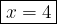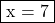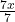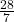## Solve the equation and enter the value of x below. 7(x + 9) + 5 = 96​

Question

Solve the equation and enter the value of x below. 7(x + 9) + 5 = 96​

in progress 0
6 months 2021-07-27T03:48:07+00:00 2 Answers 4 views 0

1. Hello!7(x + 9) + 5 = 96

Distribute:

7x + 63 + 5 = 96

Combine like terms:

7x + 68 = 96

Subtract 68 from both sides:

7x = 28

Divide both sides by 7:

x = 4Step-by-step explanation:

7(x + 9) + 5 = 96

Solve for x.

• 7(x + 9 ) + 5 = 96

Step 1 :- Distribute 7.

• 7 × x + 7 × 9 + 5 = 96
• 7x + 63 + 5 = 96

Step 2:- Add 63 and 5.

• 7x + 68 = 96

Step 3 :- Move constant to the right-hand side and change their sign.

• 7x = 96 – 68

Step 4 :- Subtract 68 from 96.

• 7x = 28

Step 5 :- Divide both side by 7.

•=• x = 4